maths > mensuration-high

Revision : Cube, Cuboid, Cylinder

what you'll learn...

Overview

Surface Area of Some Shapes: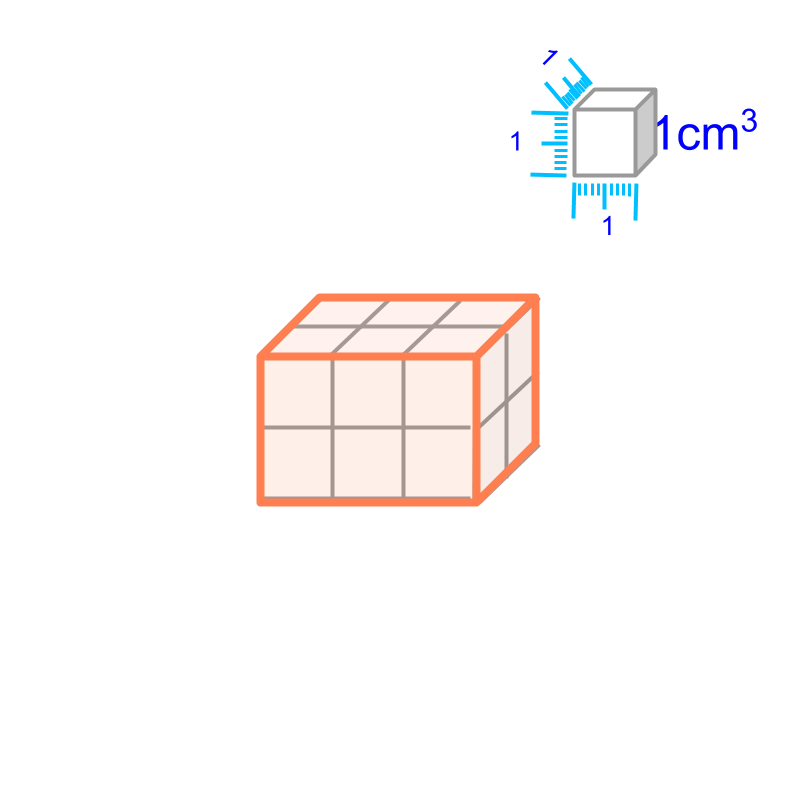Surface Area of cube $=6{q}^{2}$$= 6 {q}^{2}$

Surface Area of cuboid $=2\left(lb+bh+hl\right)$$= 2 \left(l b + b h + h l\right)$

Curved Surface Area of Cylinder $=2\pi rh$$= 2 \pi r h$

Surface Area of cylinder $=2\pi r\left(r+h\right)$$= 2 \pi r \left(r + h\right)$

surface area

The area of a shape is the "surface-span within the closed plane figure".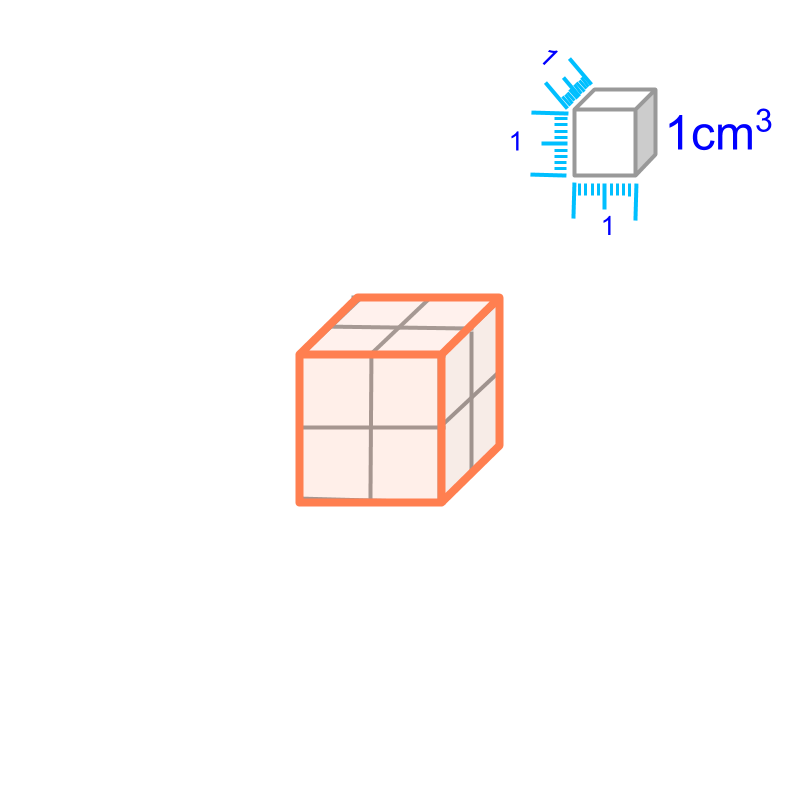A "cube" is "a 3D shape with $6$$6$ square faces".

The surface area of a cube of side $a$$a$ is "$6×{a}^{2}$$6 \times {a}^{2}$". The surface area equals the area of $6$$6$ square faces.A "cuboid" is "a 3D shape with $6$$6$ rectangular faces"

The surface area of a cuboid of length $l$$l$, breadth $b$$b$, and height $h$$h$ is "$2×\left(lb+bh+hl\right)$$2 \times \left(l b + b h + h l\right)$". The surface area equals the area of $6$$6$ rectangular faces.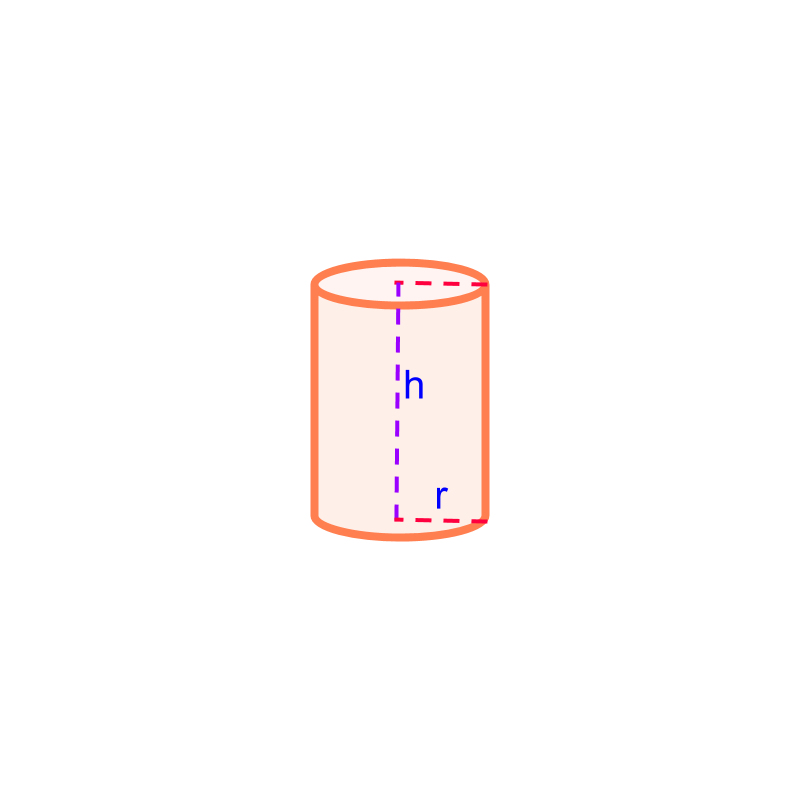Cylinder is a 3D shape that has circular cross-section uniformly along its axis.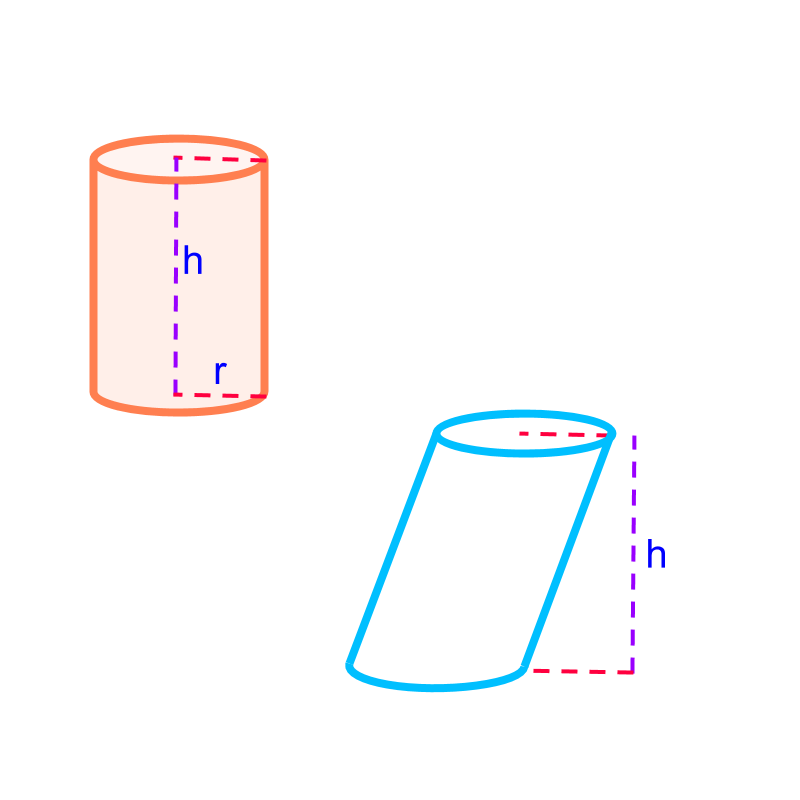When not mentioned, a cylinder is a right-cylinder with it axis at right-angle to the top and bottom faces. The other type is the oblique cylinder, in which the angle between the axis and the top (or bottom) face is not a right-angle. The right cylinder is shown in orange, and oblique cylinder is shown in blue.The surfaces in the cylinder consists of top and bottom circular-faces and a curved surface. The surface area of a cylinder of height $h$$h$ and radius $r$$r$ is the sum of the areas of ($2$$2$ circles on top and bottom) and the area of the curved surface. $=2×\pi ×{r}^{2}+2×\pi ×r×h$$= 2 \times \pi \times {r}^{2} + 2 \times \pi \times r \times h$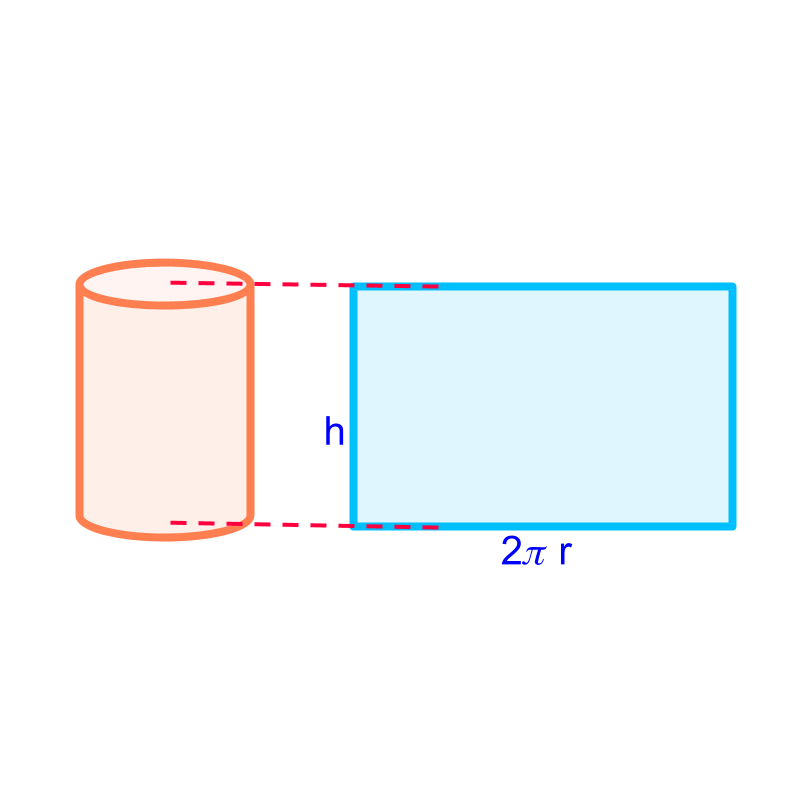Cylinder of height $h$$h$ and radius $r$$r$ is shown in the figure.

The curved surface is visualized into a rectangle of length $2\pi r$$2 \pi r$ and height $h$$h$.

The curved surface area of the cylinder equals the area of the rectangle.

Total surface area of the cylinder

$=$$=$ area of the circle on top and bottom $+$$+$ area of the curved surface

$=2×\pi ×{r}^{2}+2×\pi ×r×h$$= 2 \times \pi \times {r}^{2} + 2 \times \pi \times r \times h$

$=2\pi r\left(r+h\right)$$= 2 \pi r \left(r + h\right)$

What is the surface area of a cuboid of length $2$$2$ cm, breadth $3$$3$ cm, and height $4$$4$ cm?

The answer is "$52c{m}^{2}$$52 c {m}^{2}$"

summary

Surface Area of Some Shapes:Surface Area of cube $=6{q}^{2}$$= 6 {q}^{2}$ Surface Area of cuboid $=2\left(lb+bh+hl\right)$$= 2 \left(l b + b h + h l\right)$ Curved Surface Area of Cylinder $=2\pi rh$$= 2 \pi r h$ Surface Area of cylinder $=2\pi r\left(r+h\right)$$= 2 \pi r \left(r + h\right)$

Outline